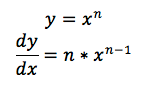### Differentiation Rules

#### Recap of Differentiation

This is all preliminary work which should have been transferred from Units 1 and 2.

###### What is Differentiation?

Differentiation measures the rate of change or gradient of a function. By determining the differentiation of an equation, you can determine the direction of the equation at a particular point, whether the equation becoming increasing positive, increasing negative or not increasing in any direction at all (stationary point).

Differentiation is usually represented as a f'(x) or dy/dx.###### Regular Differentiation RuleThe regular differentiation rule states that for a power of a variable, say x^n, the differentiation is n multiplied by x^(n-1). For example, for the equation y=5x^4, n=4 and n-1 =3, hence the differentiation is y=20x^3 (see below).